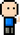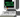# Josh Meets ComputerApril 11, 2018

# Basic Page Rank Algorithm

Algorithm can be run K times, where page rank values of each node in a network converge over time.

Steps:

1. each node in the network starts with a value of 1 / number of nodes in the network. This is their level of importance
2. for each ranking calculation, nodes pass on their current value ranking in equal parts to all their outbound connections.
3. iterating through each node in the network, each node adds to it’s own ranking the proportional ranking recieved by it’s inbound connections.
``````import networkx as nx
import matplotlib.pyplot as plt

G = nx.DiGraph()

plt.figure(figsize=(10,6))
nx.draw_networkx(G)
plt.show()``````
``````def giveRank(g, rankings, node):

# get node rank
rank = rankings[node]

# count node connections
neighbors = len(g.neighbors(node))

# divid rank by number of connections
if neighbors:
return rank / neighbors
else:
return 0

def pageRank(G, k = 1):
pageRank = {n: 0 for n in G.nodes()}

# Initialize Ranking
for n in G.nodes():
pageRank[n] += (1 / len(G.nodes()))

for _ in range(k):
curr_pageRank = pageRank
for n in G.nodes():
for neighbor in G.neighbors(n):
pageRank[n] = giveRank(G, curr_pageRank, neighbor)

return pageRank``````